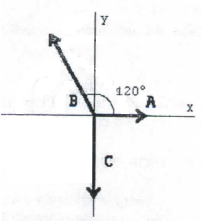# Problem: Find the magnitude and direction of the resultant R of the three vectors shown in the figure. The vectors have the following magnitudes; A = 7.9, B = 5.0, and C = 8.0. Express the direction of the vector sum by specifying the angle it makes with the positive x-axis with the counterclockwise angles taken to be positive.

###### FREE Expert Solution
88% (481 ratings)
###### Problem Details

Find the magnitude and direction of the resultant R of the three vectors shown in the figure. The vectors have the following magnitudes; A = 7.9, B = 5.0, and C = 8.0. Express the direction of the vector sum by specifying the angle it makes with the positive x-axis with the counterclockwise angles taken to be positive.Frequently Asked Questions

What scientific concept do you need to know in order to solve this problem?

Our tutors have indicated that to solve this problem you will need to apply the Trig Review concept. You can view video lessons to learn Trig Review. Or if you need more Trig Review practice, you can also practice Trig Review practice problems.

How long does this problem take to solve?

Our expert Physics tutor, Patrick took 4 minutes and 50 seconds to solve this problem. You can follow their steps in the video explanation above.

What professor is this problem relevant for?

Based on our data, we think this problem is relevant for Professor Krasnoslobodtsev's class at UN OMAHA.